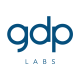310

# ECML PKDD 2017October 25, 2017

## Transcript

1. Towards True End-to-End
Learning and Optimization
michell.s.handaka [at] gdplabs.id
GDP Labs Confidential

2. GDP Labs Confidential

3. GDP Labs Confidential

4. Deep Learning
learns features from data
GDP Labs Confidential

5. Deep Learning
learns features from data
GDP Labs Confidential

6. Deep Learning
learns features from data
GDP Labs Confidential

7. Deep Learning
end-to-end learning: joint optimization of a single loss function
GDP Labs Confidential

8. Deep Learning
end-to-end learning: joint optimization of a single loss function
GDP Labs Confidential

9. Towards True End-to-End Learning and Optimization
Deep Learning
AutoML
deep learning
“end-to-end”
expert chooses
architecture & hyperparameters
learning
box
meta-level
learning and optimization
GDP Labs Confidential

10. Learning Box can be Any Machine Learning Pipeline
● data preprocessing
● feature engineering
● model selection
● hyperparameter tuning
● ensembles
GDP Labs Confidential

11. Bayesian Optimization
Bayesian
optimization
λ
f(λ)
GDP Labs Confidential

12. GDP Labs Confidential

13. GDP Labs Confidential

14. GDP Labs Confidential

15. [Bardenet et al., ICML 2013; Swersky et al., NIPS 2013;
Feurer, Springenberg, Hutter, AAAI 2015 ]
[Domhan, Springenberg, Hutter, IJCAI 2015]
[Klein, Bartels, Falkner, Hennig, Hutter, AISTATS 2017]
Beyond Black Box Bayesian Optimization
[Thornton, Hutter, Hoos, Leyton-Brown, KDD 2013]
GDP Labs Confidential

16. Speeding Up Automatic Hyperparameter Optimization of
Deep Neural Networks by Extrapolation of Learning Curves
[Domhan, Springenberg, Hutter, IJCAI 2015]
GDP Labs Confidential

17. Typical Learning Curves for Iterative Training with SGD
Markov Chain Monte Carlo to quantify
model uncertainty
GDP Labs Confidential

18. Predictive Termination
if P < 5%, terminate
if P > 5%, continue training
GDP Labs Confidential

19. Qualitative Analysis
GDP Labs Confidential

20. Quantitative Analysis
2 fold speed up of Deep Neural Network
structure & hyperparameter optimization
GDP Labs Confidential

21. Fast Bayesian Optimization of
Machine Learning Hyperparameters on Large Datasets
[Klein, Bartels, Falkner, Hennig, Hutter, AISTATS 2017]
GDP Labs Confidential

22. problem: training is very slow for large datasets
approach: scaling up from subsets of the data
e.g. Support Vector Machine
computational cost grows quadratically in dataset size s
error shrinks smoothly with dataset size s
GDP Labs Confidential

23. ● automatically choose dataset size for each evaluation
● entropy search based on a probability distribution of where the maximum lies
● pick configuration and dataset size pair to maximally decrease entropy per time spent
GDP Labs Confidential

24. ● 10 - 500 fold speed up for optimizing
SVMs
● 5 - 10 fold speed up for optimizing
Convolutional Neural Networks
Quantitative Analysis
GDP Labs Confidential

25. Bayesian Optimization with
Robust Bayesian Neural Networks
[Springenberg, Klein, Falkner, Hutter, NIPS 2016]
GDP Labs Confidential

26. f(λ, D) ✓ f(λ, t) ✓ f(λ, s) ✓
f(λ, D, t, s)?
● a lot of data points
● expensive black box evaluations
● cheap incremental evaluations
● Gaussian Process Model will not scale
GDP Labs Confidential

27. Empirical Evaluation
Scalable Bayesian Optimization Using
Deep Neural Networks (DNGO)
[Snoek et al., ICML 2015]
DNN with Bayesian Linear Regression in
last layer
both algorithms are effective
SGHMC is more robust
as good as Bayesian optimization with
Gaussian Processes but much more flexible
e.g. reasoning over many related datasets
GDP Labs Confidential

28. Conclusion
● Bayesian optimization enables true end-to-end learning
● large speed ups by going beyond black box optimization
● learning across datasets
● learning curve extrapolation
● dataset subsampling
GDP Labs Confidential

29. References
● Domhan et al. Speeding up automatic hyperparameter optimization of deep neural networks by extrapolation of learning curves. IJCAI 2015.
● Klein et al. Fast Bayesian optimization of machine learning hyperparameters on large datasets. AISTATS 2017.
● Springenberg. Bayesian optimization with robust Bayesian neural networks. NIPS 2016.
● Snoek et al. Scalable Bayesian optimization using deep neural networks. ICML 2015.
● Hutter. Towards true end-to-end learning and optimization. ECML 2017.
● Hutter. Black box hyperparameter optimization and AutoML. AutoML 2017.
● Hutter. Beyond black box optimization. AutoML 2017.